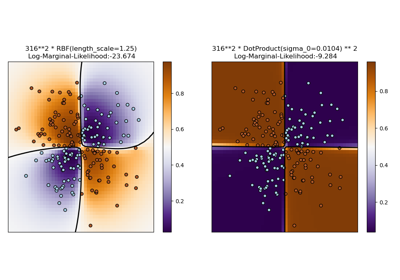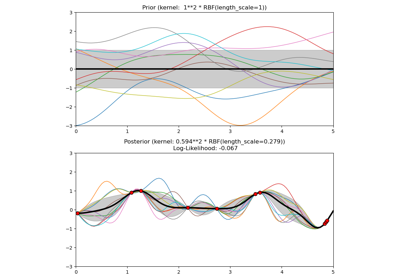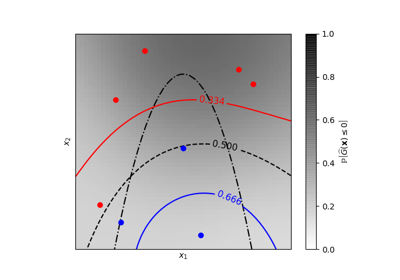# sklearn.gaussian_process.kernels.DotProduct¶

class sklearn.gaussian_process.kernels.DotProduct(sigma_0=1.0, sigma_0_bounds=(1e-05, 100000.0))[source]

Dot-Product kernel.

The DotProduct kernel is non-stationary and can be obtained from linear regression by putting $$N(0, 1)$$ priors on the coefficients of $$x_d (d = 1, . . . , D)$$ and a prior of $$N(0, \sigma_0^2)$$ on the bias. The DotProduct kernel is invariant to a rotation of the coordinates about the origin, but not translations. It is parameterized by a parameter sigma_0 $$\sigma$$ which controls the inhomogenity of the kernel. For $$\sigma_0^2 =0$$, the kernel is called the homogeneous linear kernel, otherwise it is inhomogeneous. The kernel is given by

$k(x_i, x_j) = \sigma_0 ^ 2 + x_i \cdot x_j$

The DotProduct kernel is commonly combined with exponentiation.

See , Chapter 4, Section 4.2, for further details regarding the DotProduct kernel.

Read more in the User Guide.

New in version 0.18.

Parameters
sigma_0float >= 0, default=1.0

Parameter controlling the inhomogenity of the kernel. If sigma_0=0, the kernel is homogenous.

sigma_0_boundspair of floats >= 0 or “fixed”, default=(1e-5, 1e5)

The lower and upper bound on ‘sigma_0’. If set to “fixed”, ‘sigma_0’ cannot be changed during hyperparameter tuning.

Attributes
bounds

Returns the log-transformed bounds on the theta.

hyperparameter_sigma_0
hyperparameters

Returns a list of all hyperparameter specifications.

n_dims

Returns the number of non-fixed hyperparameters of the kernel.

requires_vector_input

Returns whether the kernel is defined on fixed-length feature vectors or generic objects.

theta

Returns the (flattened, log-transformed) non-fixed hyperparameters.

References

1

Carl Edward Rasmussen, Christopher K. I. Williams (2006). “Gaussian Processes for Machine Learning”. The MIT Press.

Examples

>>> from sklearn.datasets import make_friedman2
>>> from sklearn.gaussian_process import GaussianProcessRegressor
>>> from sklearn.gaussian_process.kernels import DotProduct, WhiteKernel
>>> X, y = make_friedman2(n_samples=500, noise=0, random_state=0)
>>> kernel = DotProduct() + WhiteKernel()
>>> gpr = GaussianProcessRegressor(kernel=kernel,
...         random_state=0).fit(X, y)
>>> gpr.score(X, y)
0.3680...
>>> gpr.predict(X[:2,:], return_std=True)
(array([653.0..., 592.1...]), array([316.6..., 316.6...]))


Methods

 __call__(X[, Y, eval_gradient]) Return the kernel k(X, Y) and optionally its gradient. clone_with_theta(theta) Returns a clone of self with given hyperparameters theta. Returns the diagonal of the kernel k(X, X). get_params([deep]) Get parameters of this kernel. Returns whether the kernel is stationary. set_params(**params) Set the parameters of this kernel.
__init__(sigma_0=1.0, sigma_0_bounds=(1e-05, 100000.0))[source]

Initialize self. See help(type(self)) for accurate signature.

__call__(X, Y=None, eval_gradient=False)[source]

Return the kernel k(X, Y) and optionally its gradient.

Parameters
Xndarray of shape (n_samples_X, n_features)

Left argument of the returned kernel k(X, Y)

Yndarray of shape (n_samples_Y, n_features), default=None

Right argument of the returned kernel k(X, Y). If None, k(X, X) if evaluated instead.

Determines whether the gradient with respect to the kernel hyperparameter is determined. Only supported when Y is None.

Returns
Kndarray of shape (n_samples_X, n_samples_Y)

Kernel k(X, Y)

K_gradientndarray of shape (n_samples_X, n_samples_X, n_dims), optional

The gradient of the kernel k(X, X) with respect to the hyperparameter of the kernel. Only returned when eval_gradient is True.

property bounds

Returns the log-transformed bounds on the theta.

Returns
boundsndarray of shape (n_dims, 2)

The log-transformed bounds on the kernel’s hyperparameters theta

clone_with_theta(theta)[source]

Returns a clone of self with given hyperparameters theta.

Parameters
thetandarray of shape (n_dims,)

The hyperparameters

diag(X)[source]

Returns the diagonal of the kernel k(X, X).

The result of this method is identical to np.diag(self(X)); however, it can be evaluated more efficiently since only the diagonal is evaluated.

Parameters
Xndarray of shape (n_samples_X, n_features)

Left argument of the returned kernel k(X, Y).

Returns
K_diagndarray of shape (n_samples_X,)

Diagonal of kernel k(X, X).

get_params(deep=True)[source]

Get parameters of this kernel.

Parameters
deepbool, default=True

If True, will return the parameters for this estimator and contained subobjects that are estimators.

Returns
paramsdict

Parameter names mapped to their values.

property hyperparameters

Returns a list of all hyperparameter specifications.

is_stationary()[source]

Returns whether the kernel is stationary.

property n_dims

Returns the number of non-fixed hyperparameters of the kernel.

property requires_vector_input

Returns whether the kernel is defined on fixed-length feature vectors or generic objects. Defaults to True for backward compatibility.

set_params(**params)[source]

Set the parameters of this kernel.

The method works on simple kernels as well as on nested kernels. The latter have parameters of the form <component>__<parameter> so that it’s possible to update each component of a nested object.

Returns
self
property theta

Returns the (flattened, log-transformed) non-fixed hyperparameters.

Note that theta are typically the log-transformed values of the kernel’s hyperparameters as this representation of the search space is more amenable for hyperparameter search, as hyperparameters like length-scales naturally live on a log-scale.

Returns
thetandarray of shape (n_dims,)

The non-fixed, log-transformed hyperparameters of the kernel

## Examples using sklearn.gaussian_process.kernels.DotProduct¶